# Multiplication and Division

There are many strategies that can be employed to multiply and divide larger numbers. Students will deepen their conceptual knowledge of multiplication and division, starting with visual models like arrays and diagrams.Then students will then move to more abstract calculation methods like partial products, the distributive property and standard algorithms.
This lesson includes 5 printable learning activities.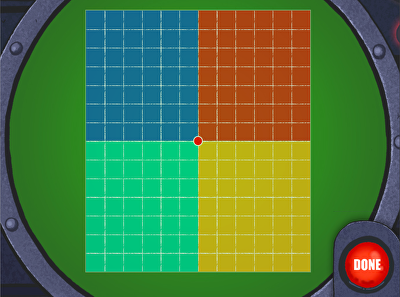The Muggos' radar has gone haywire! Kids help the fleet get back on course by calculating the products of different arrays. Perfect for practise counting up units by column and row, this game helps kids visualize the array strategy for solving bigger two-digit multiplication problems. After solving smaller arrays, kids use addition to find the product of the larger, total array.

## Multi-Digit Multiplication and Partial Products 1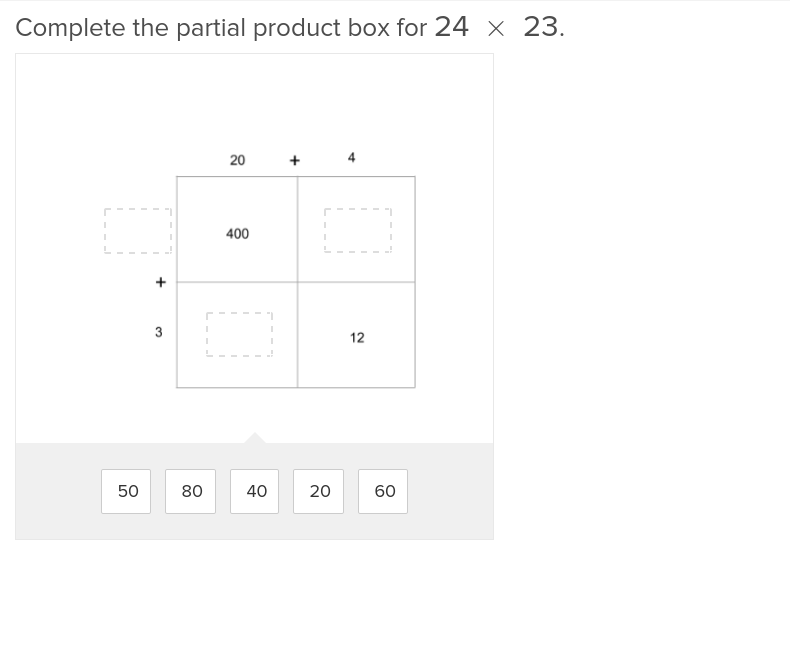Break down multi-digit multiplication with these exercises that lead students step-by-step through the partial product method.

## Multi-Digit Multiplication and the Standard Algorithm 1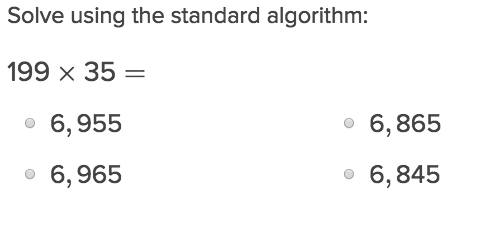Introduce students to multi digit multiplication within standard algorithms using this helpful exercise from Education.com.

## Distributive Property of MultiplicationHelp fourth graders solidify their knowledge of multiplication by teaching them the distributive property and how it can aid them in solving problems.

## Division without Remainders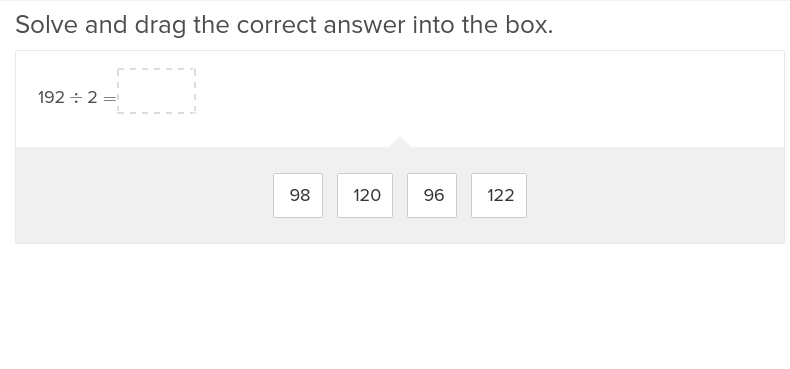Before students can move on to more complicated matters, first help them understand division without remainders.

## Division with Remainders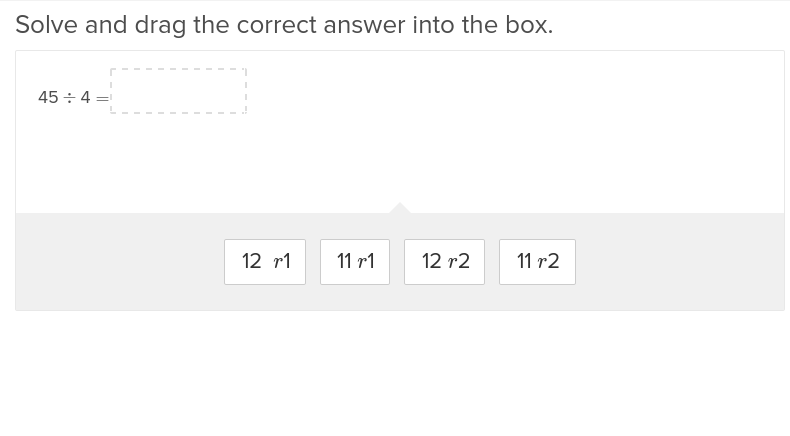When students are ready for a challenge, assign this exercise that introduces division with remainders.

## Divisibility RulesTeach students divisibility rules the easy way with this easily navigated interactive exercise from Education.com.

Create new collection

0

### New Collection>

0Items

What could we do to improve Education.com?